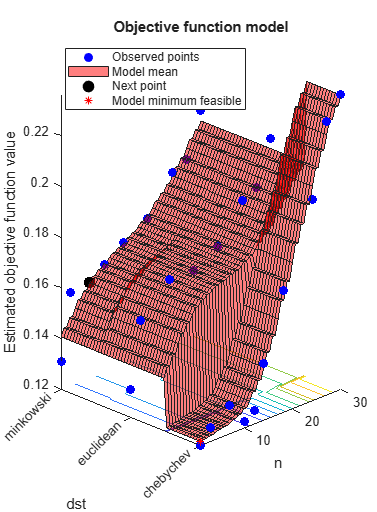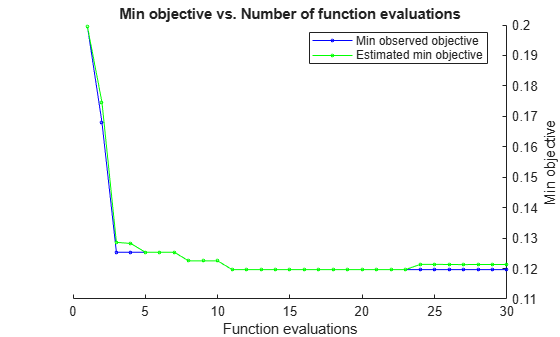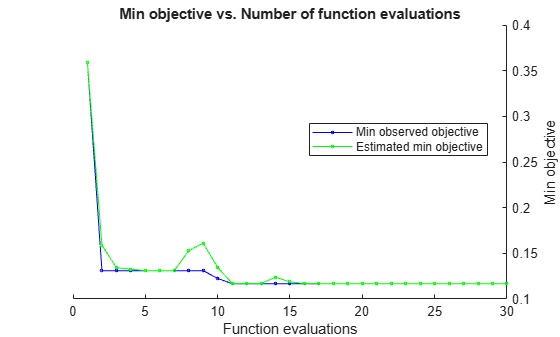# BayesianOptimization

Bayesian optimization results

## Description

A `BayesianOptimization` object contains the results of a Bayesian optimization. It is the output of `bayesopt` or a fit function that accepts the `OptimizeHyperparameters` name-value pair such as `fitcdiscr`. In addition, a `BayesianOptimization` object contains data for each iteration of `bayesopt` that can be accessed by a plot function or an output function.

## Creation

Create a `BayesianOptimization` object using the `bayesopt` function or a fit function with the `OptimizeHyperparameters` name-value pair.

## Properties

expand all

### Problem Definition Properties

`ObjectiveFcn` argument used by `bayesopt`, returned as a function handle.

• If you call `bayesopt` directly, `ObjectiveFcn` is the `bayesopt` objective function argument.

• If you call a fit function containing the `'OptimizeHyperparameters'` name-value pair argument, `ObjectiveFcn` is a function handle that returns the misclassification rate for classification or returns the logarithm of one plus the cross-validation loss for regression, measured by five-fold cross-validation.

Data Types: `function_handle`

`VariableDescriptions` argument that `bayesopt` used, returned as a vector of `optimizableVariable` objects.

• If you called `bayesopt` directly, `VariableDescriptions` is the `bayesopt` variable description argument.

• If you called a fit function with the `OptimizeHyperparameters` name-value pair, `VariableDescriptions` is the vector of hyperparameters.

Options that `bayesopt` used, returned as a structure.

• If you called `bayesopt` directly, `Options` is the options used in `bayesopt`, which are the name-value pairs See `bayesopt` Input Arguments.

• If you called a fit function with the `OptimizeHyperparameters` name-value pair, `Options` are the default `bayesopt` options, modified by the `HyperparameterOptimizationOptions` name-value pair.

`Options` is a read-only structure containing the following fields.

Option NameMeaning
`AcquisitionFunctionName`Acquisition function name. See Acquisition Function Types.
`IsObjectiveDeterministic``true` means the objective function is deterministic, `false` otherwise.
`ExplorationRatio`Used only when `AcquisitionFunctionName` is `'expected-improvement-plus'` or `'expected-improvement-per-second-plus'`. See Plus.

`MaxObjectiveEvaluations`Objective function evaluation limit.
`MaxTime`Time limit.

`XConstraintFcn`Deterministic constraints on variables. See Deterministic Constraints — XConstraintFcn.
`ConditionalVariableFcn`Conditional variable constraints. See Conditional Constraints — ConditionalVariableFcn.
`NumCoupledConstraints`Number of coupled constraints. See Coupled Constraints.
`CoupledConstraintTolerances`Coupled constraint tolerances. See Coupled Constraints.
`AreCoupledConstraintsDeterministic`Logical vector specifying whether each coupled constraint is deterministic.

`Verbose`Command-line display level.
`OutputFcn`Function called after each iteration. See Bayesian Optimization Output Functions.
`SaveVariableName`Variable name for the `@assignInBase` output function.
`SaveFileName`File name for the `@saveToFile` output function.
`PlotFcn`Plot function called after each iteration. See Bayesian Optimization Plot Functions

`InitialX`Points where `bayesopt` evaluated the objective function.
`InitialObjective`Objective function values at `InitialX`.
`InitialConstraintViolations`Coupled constraint function values at `InitialX`.
`InitialErrorValues`Error values at `InitialX`.
`InitialObjectiveEvaluationTimes`Objective function evaluation times at `InitialX`.
`InitialIterationTimes`Time for each iteration, including objective function evaluation and other computations.

Data Types: `struct`

### Solution Properties

Minimum observed value of objective function, returned as a real scalar. When there are coupled constraints or evaluation errors, this value is the minimum over all observed points that are feasible according to the final constraint and Error models.

Data Types: `double`

Observed point with minimum objective function value, returned as a `1`-by-`D` table, where `D` is the number of variables.

Data Types: `table`

Minimum estimated value of objective function, returned as a real scalar. `MinEstimatedObjective` uses the final objective model.

`MinEstimatedObjective` is the same as the `CriterionValue` result of `bestPoint` with default criterion.

Data Types: `double`

Point with minimum estimated objective function value, returned as a `1`-by-`D` table, where `D` is the number of variables. `XAtMinEstimatedObjective` uses the final objective model.

Data Types: `table`

Number of objective function evaluations, returned as a positive integer. This includes the initial evaluations to form a posterior model as well as evaluation during the optimization iterations.

Data Types: `double`

Total elapsed time of optimization in seconds, returned as a positive scalar.

Data Types: `double`

Next point to evaluate if optimization continues, returned as a `1`-by-`D` table, where `D` is the number of variables.

Data Types: `table`

### Trace Properties

Points where the objective function was evaluated, returned as a `T`-by-`D` table, where `T` is the number of evaluation points and `D` is the number of variables.

Data Types: `table`

Objective function values, returned as a column vector of length `T`, where `T` is the number of evaluation points. `ObjectiveTrace` contains the history of objective function evaluations.

Data Types: `double`

Objective function evaluation times, returned as a column vector of length `T`, where `T` is the number of evaluation points. `ObjectiveEvaluationTimeTrace` includes the time in evaluating coupled constraints, because the objective function computes these constraints.

Data Types: `double`

Iteration times, returned as a column vector of length `T`, where `T` is the number of evaluation points. `IterationTimeTrace` includes both objective function evaluation time and other overhead.

Data Types: `double`

Coupled constraint values, returned as a `T`-by-`K` array, where `T` is the number of evaluation points and `K` is the number of coupled constraints.

Data Types: `double`

Error indications, returned as a column vector of length `T` of `-1` or `1` entries, where `T` is the number of evaluation points. Each `1` entry indicates that the objective function errored or returned `NaN` on the corresponding point in `XTrace`. Each `-1` entry indicates that the objective function value was computed.

Data Types: `double`

Feasibility indications, returned as a logical column vector of length `T`, where `T` is the number of evaluation points. Each `1` entry indicates that the final constraint model predicts feasibility at the corresponding point in `XTrace`.

Data Types: `logical`

Probability that evaluation point is feasible, returned as a column vector of length `T`, where `T` is the number of evaluation points. The probabilities come from the final constraint model, including the error constraint model, on the corresponding points in `XTrace`.

Data Types: `double`

Which evaluation gave minimum feasible objective, returned as a column vector of integer indices of length `T`, where `T` is the number of evaluation points. Feasibility is determined with respect to the constraint models that existed at each iteration, including the error constraint model.

Data Types: `double`

Minimum observed objective, returned as a column vector of integer indices of length `T`, where `T` is the number of evaluation points.

Data Types: `double`

Minimum estimated objective, returned as a column vector of integer indices of length `T`, where `T` is the number of evaluation points. The estimated objective at each iteration is determined with respect to the objective model that existed at that iteration.

Data Types: `double`

Auxiliary data from the objective function, returned as a cell array of length `T`, where `T` is the number of evaluation points. Each entry in the cell array is the `UserData` returned in the third output of the objective function.

Data Types: `cell`

## Object Functions

 `bestPoint` Best point in a Bayesian optimization according to a criterion `plot` Plot Bayesian optimization results `predictConstraints` Predict coupled constraint violations at a set of points `predictError` Predict error value at a set of points `predictObjective` Predict objective function at a set of points `predictObjectiveEvaluationTime` Predict objective function run times at a set of points `resume` Resume a Bayesian optimization

## Examples

collapse all

This example shows how to create a `BayesianOptimization` object by using `bayesopt` to minimize cross-validation loss.

Optimize hyperparameters of a KNN classifier for the `ionosphere` data, that is, find KNN hyperparameters that minimize the cross-validation loss. Have `bayesopt` minimize over the following hyperparameters:

• Nearest-neighborhood sizes from 1 to 30

• Distance functions `'chebychev'`, `'euclidean'`, and `'minkowski'`.

For reproducibility, set the random seed, set the partition, and set the `AcquisitionFunctionName` option to `'expected-improvement-plus'`. To suppress iterative display, set `'Verbose'` to `0`. Pass the partition `c` and fitting data `X` and `Y` to the objective function `fun` by creating `fun` as an anonymous function that incorporates this data. See Parameterizing Functions.

```load ionosphere rng default num = optimizableVariable('n',[1,30],'Type','integer'); dst = optimizableVariable('dst',{'chebychev','euclidean','minkowski'},'Type','categorical'); c = cvpartition(351,'Kfold',5); fun = @(x)kfoldLoss(fitcknn(X,Y,'CVPartition',c,'NumNeighbors',x.n,... 'Distance',char(x.dst),'NSMethod','exhaustive')); results = bayesopt(fun,[num,dst],'Verbose',0,... 'AcquisitionFunctionName','expected-improvement-plus')``````results = BayesianOptimization with properties: ObjectiveFcn: [function_handle] VariableDescriptions: [1x2 optimizableVariable] Options: [1x1 struct] MinObjective: 0.1197 XAtMinObjective: [1x2 table] MinEstimatedObjective: 0.1213 XAtMinEstimatedObjective: [1x2 table] NumObjectiveEvaluations: 30 TotalElapsedTime: 91.4349 NextPoint: [1x2 table] XTrace: [30x2 table] ObjectiveTrace: [30x1 double] ConstraintsTrace: [] UserDataTrace: {30x1 cell} ObjectiveEvaluationTimeTrace: [30x1 double] IterationTimeTrace: [30x1 double] ErrorTrace: [30x1 double] FeasibilityTrace: [30x1 logical] FeasibilityProbabilityTrace: [30x1 double] IndexOfMinimumTrace: [30x1 double] ObjectiveMinimumTrace: [30x1 double] EstimatedObjectiveMinimumTrace: [30x1 double] ```

This example shows how to minimize the cross-validation loss in the `ionosphere` data using Bayesian optimization of an SVM classifier.

`load ionosphere`

Optimize the classification using the `'auto'` parameters.

```rng default % For reproducibility Mdl = fitcsvm(X,Y,'OptimizeHyperparameters','auto')```
```|=====================================================================================================| | Iter | Eval | Objective | Objective | BestSoFar | BestSoFar | BoxConstraint| KernelScale | | | result | | runtime | (observed) | (estim.) | | | |=====================================================================================================| | 1 | Best | 0.21937 | 23.337 | 0.21937 | 0.21937 | 64.836 | 0.0015729 | | 2 | Accept | 0.35897 | 0.30186 | 0.21937 | 0.22807 | 0.036335 | 5.5755 | | 3 | Best | 0.13105 | 13.254 | 0.13105 | 0.14149 | 0.0022147 | 0.0023957 | | 4 | Accept | 0.35897 | 0.31133 | 0.13105 | 0.13108 | 5.1259 | 98.62 | | 5 | Best | 0.12821 | 0.23976 | 0.12821 | 0.12823 | 0.0010294 | 0.03159 | | 6 | Accept | 0.1396 | 0.56146 | 0.12821 | 0.13068 | 0.0011115 | 0.0086377 | | 7 | Accept | 0.13105 | 2.0337 | 0.12821 | 0.13004 | 0.022744 | 0.019059 | | 8 | Accept | 0.1339 | 0.3798 | 0.12821 | 0.13101 | 0.0010632 | 0.03521 | | 9 | Accept | 0.1339 | 45.932 | 0.12821 | 0.13093 | 4.3141 | 0.032466 | | 10 | Best | 0.12536 | 0.64057 | 0.12536 | 0.12563 | 0.001052 | 0.022689 | | 11 | Best | 0.12251 | 0.5482 | 0.12251 | 0.12414 | 0.0010061 | 0.023339 | | 12 | Accept | 0.12251 | 1.2241 | 0.12251 | 0.12352 | 0.0011285 | 0.020533 | | 13 | Accept | 0.1396 | 0.8759 | 0.12251 | 0.12359 | 0.0010288 | 0.22378 | | 14 | Accept | 0.12251 | 0.64389 | 0.12251 | 0.12374 | 0.0074141 | 0.11729 | | 15 | Accept | 0.12536 | 0.96902 | 0.12251 | 0.12379 | 0.097268 | 0.16841 | | 16 | Accept | 0.13105 | 1.0576 | 0.12251 | 0.12368 | 0.10661 | 0.1072 | | 17 | Accept | 0.1396 | 0.22017 | 0.12251 | 0.12582 | 0.0010783 | 0.12884 | | 18 | Accept | 0.12536 | 0.18624 | 0.12251 | 0.12545 | 0.0011004 | 0.020963 | | 19 | Accept | 0.12251 | 0.25348 | 0.12251 | 0.12354 | 0.001037 | 0.020043 | | 20 | Accept | 0.12251 | 0.18241 | 0.12251 | 0.12356 | 0.014246 | 0.18936 | |=====================================================================================================| | Iter | Eval | Objective | Objective | BestSoFar | BestSoFar | BoxConstraint| KernelScale | | | result | | runtime | (observed) | (estim.) | | | |=====================================================================================================| | 21 | Accept | 0.12536 | 0.35297 | 0.12251 | 0.1237 | 0.016633 | 0.14997 | | 22 | Accept | 0.12251 | 0.20078 | 0.12251 | 0.12365 | 0.034052 | 0.30274 | | 23 | Accept | 0.12821 | 0.2307 | 0.12251 | 0.1239 | 0.029199 | 0.23923 | | 24 | Accept | 0.1339 | 0.18804 | 0.12251 | 0.12402 | 0.10543 | 0.34155 | | 25 | Accept | 0.12251 | 0.15935 | 0.12251 | 0.1232 | 0.02079 | 0.19576 | | 26 | Accept | 0.12821 | 0.26573 | 0.12251 | 0.12405 | 0.019174 | 0.19892 | | 27 | Accept | 0.1396 | 0.15935 | 0.12251 | 0.12364 | 0.037809 | 1.2333 | | 28 | Accept | 0.1396 | 0.15872 | 0.12251 | 0.12368 | 0.010133 | 0.67582 | | 29 | Accept | 0.12536 | 0.19032 | 0.12251 | 0.12372 | 0.35714 | 0.9077 | | 30 | Accept | 0.12251 | 0.22739 | 0.12251 | 0.12375 | 1.7269 | 0.95511 | ``````__________________________________________________________ Optimization completed. MaxObjectiveEvaluations of 30 reached. Total function evaluations: 30 Total elapsed time: 217.4842 seconds Total objective function evaluation time: 95.2856 Best observed feasible point: BoxConstraint KernelScale _____________ ___________ 0.0010061 0.023339 Observed objective function value = 0.12251 Estimated objective function value = 0.12425 Function evaluation time = 0.5482 Best estimated feasible point (according to models): BoxConstraint KernelScale _____________ ___________ 0.0011004 0.020963 Estimated objective function value = 0.12375 Estimated function evaluation time = 0.43825 ```
```Mdl = ClassificationSVM ResponseName: 'Y' CategoricalPredictors: [] ClassNames: {'b' 'g'} ScoreTransform: 'none' NumObservations: 351 HyperparameterOptimizationResults: [1x1 BayesianOptimization] Alpha: [94x1 double] Bias: -5.4507 KernelParameters: [1x1 struct] BoxConstraints: [351x1 double] ConvergenceInfo: [1x1 struct] IsSupportVector: [351x1 logical] Solver: 'SMO' Properties, Methods ```

The fit achieved about 12% loss for the default 5-fold cross validation.

Examine the `BayesianOptimization` object that is returned in the `HyperparameterOptimizationResults` property of the returned model.

`disp(Mdl.HyperparameterOptimizationResults)`
``` BayesianOptimization with properties: ObjectiveFcn: @createObjFcn/inMemoryObjFcn VariableDescriptions: [5x1 optimizableVariable] Options: [1x1 struct] MinObjective: 0.1225 XAtMinObjective: [1x2 table] MinEstimatedObjective: 0.1237 XAtMinEstimatedObjective: [1x2 table] NumObjectiveEvaluations: 30 TotalElapsedTime: 217.4842 NextPoint: [1x2 table] XTrace: [30x2 table] ObjectiveTrace: [30x1 double] ConstraintsTrace: [] UserDataTrace: {30x1 cell} ObjectiveEvaluationTimeTrace: [30x1 double] IterationTimeTrace: [30x1 double] ErrorTrace: [30x1 double] FeasibilityTrace: [30x1 logical] FeasibilityProbabilityTrace: [30x1 double] IndexOfMinimumTrace: [30x1 double] ObjectiveMinimumTrace: [30x1 double] EstimatedObjectiveMinimumTrace: [30x1 double] ```